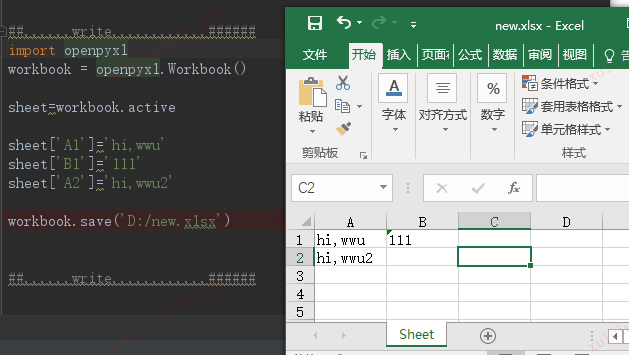# python如何读写excel文件

python读写excel的方式有很多，不同的模块在读写的方法上稍有区别：

# Python使用openpyxl读写excel文件

 姓名 性别 张三 男 李四 女

`#coding=gbk`

# 获得所有sheet的名称
print(wb.get_sheet_names())
# 根据sheet名字获得sheet
a_sheet = wb.get_sheet_by_name('Sheet1')
# 获得sheet名
print(a_sheet.title)
# 获得当前正在显示的sheet, 也可以用wb.get_active_sheet()
sheet = wb.active

# 获取某个单元格的值，观察excel发现也是先字母再数字的顺序，即先列再行

```b3 = worksheet['B3']
print('B3是----',b3) # 返回的数字就是int型
print(f'({b3.column}, {b3.row}) is {b3.value}') # 返回的数字就是int型```

B3是---- <Cell 'Sheet1'.B3>
(2, 3) is 女

```cell_2_2=worksheet.cell(row=2,column=2)
cell_2_2_value=worksheet.cell(row=2,column=2).value
print('第2行第2列',cell_2_2)
print('第2行第2列值',cell_2_2_value)```

# 获得最大列和最大行

```print('最大行数---', worksheet.max_row)
print('最大列数---', worksheet.max_column)```

```row3=[item.value for item in list(worksheet.rows)]
print('第3行值',row3)
col2=[item.value for item in list(worksheet.columns)]
print('第2列值',col2)```

```# print('通过worksheet.cell获取所有数据方法一：')
# for i in range(1, 4):
#     for j in range(1, 3):
#         print(worksheet.cell(row=i, column=j))

print('通过worksheet.cell获取所有数据方法二：')
for row_cell in worksheet['A1':'B3']:
for cell in row_cell:
print(cell)```

<Cell 'Sheet1'.A1>
<Cell 'Sheet1'.B1>
<Cell 'Sheet1'.A2>
<Cell 'Sheet1'.B2>
<Cell 'Sheet1'.A3>
<Cell 'Sheet1'.B3>

from openpyxl import Workbook
wb = Workbook()

print(wb.get_sheet_names()) # 提供一个默认名叫Sheet的表，office2016下新建提供默认Sheet1
# 直接赋值就可以改工作表的名称
sheet.title = 'Sheet1'
# 新建一个工作表，可以指定索引，适当安排其在工作簿中的位置
wb.create_sheet('Data', index=1) # 被安排到第二个工作表，index=0就是第一个位置
# 删除某个工作表
wb.remove(sheet)
del wb[sheet]

# 直接给单元格赋值就行
sheet['A1'] = 'good'
# B9处写入平均值
sheet['B9'] = '=AVERAGE(B2:B8)'

wb.save(r'D:\example.xlsx')wb.save(r'D:\example.xlsx')